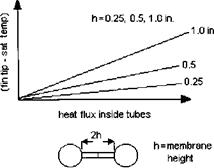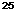## Energy Absorbed by the Furnace

The energy transferred to the furnace is obtained from the equation Q = — T4) = Wf LHV — Wghe^ ^ heat 1lux Figure 3.11 Relating fin tip temperature to heat flux in membrane wall furnace.FIgure 3.12 Furnace outlet temperature for gas and oil firing.

Where

Q = energy transferred to the furnace, Btu/h Tg = average gas temperature in the furnace, °R he = enthalpy of flue gases corresponding to the furnace exit gas temperature Te, Btu/lb Tw = average furnace wall temperature, °R Ap = effective projected area of the furnace, ft2 a = radiation constant e1, e2 = emissivity of flame and wall, respectively LHV = lower heating value of the fuel, Btu/lb Wf, Wg = fuel and flue gas quantity, lb/h

The emissivity of the flame may be determined by using methods discussed in Q8.08. The effective projected area includes the water-cooled surfaces and the opening to the furnace exit plane. If refractory is used on part of the surfaces, a correction factor of 0.3-0.5 has to be used for its effectiveness. Once the furnace duty is arrived at, the heat flux inside the tube may be estimated. Heat flux inside the tubes is a very important parameter because it affects the boiling process.

Example 4

Determine the energy absorbed by the packaged boiler furnace firing natural gas for which data are given in Table 3.4. At 100% load, boiler duty or energy absorbed by steam = 118.71 MM Btu/h. Flue gas flow = 125,246 lb/h at 100% load.

TABLE 3.4 Boiler Performance—Gas Firing3

 2550 75 100

 Boiler duty, MM Btu/h 29.14 Excess air, % 30 Fuel input, MM Btu/h 34.68 Heat rel rate, Btu/ft3 h 16,055 Heat rel rate, Btu/ft2 h 29,646 Steam flow, lb/h 25,000 Steam temperature, °F 711 Economizer exit water 328 Temp, °F Boiler exit gas temp, °F 525 Economizer exit gas 254 Temp, °F Air flow, lb/h 32,954 Flue gas, lb/h 34,413 Dry gas loss, % 3.71 Air moisture loss, % 0.1 Fuel moisture loss, % 10.48 Casing loss, % 1.2 Margin, % 0.5 Efficiency, % HHV 84.01 Efficiency, % LHV 93.12 Furnace back pressure, 0.8
 In. WC

 50.09 89.03 118.71 15 15 15 69.79 105.69 141.89 32,310 48,931 65,691 59,660 90,349 121,297 50,000 75,000 100,000 740 750 750 334 356 374 587 666 739 271 298 327 58,665 88,843 119,275 61,602 93,290 125,246 3.58 4.08 4.62 0.1 0.1 0.12 10.55 10.67 10.79 0.6 0.4 0.3 0.5 0.5 0.5 84.67 84.24 83.66 93.85 93.37 92.73 2.61 6.21 11.49

ASteam pressure 500 psig; feedwater 230°F, blowdown 1%, amb temp 80°F; RH 60%, fuel — standard natural gas. Flue gas analysis (vol%): CO2 = 8.29, H2O = 18.17, N2 = 71,

0. 07, O2 = 2.46. Boiler furnace projected area = 1169 ft2, furnace width = 7.5 ft, length = 32 ft, height = 9 ft.

The net heat input to the furnace is

0 992

118.71 x ——— = 127 MM Btu/h

0. 9273 1

Where 0.992 = 1 — heat losses, and 0.9273 is the boiler efficiency on LHV basis. Net heat input 127 x 106

= 108,900 Btu/ft2 h

Effective furnace area 1169

The furnace exit gas temperature from Fig. 3.12 Is 2235°F. It may be shown that the enthalpy of the flue gases at 2235°F is 661.4 Btu/lb based on the flue gas analysis. (See Appendix, Table A8.)

The furnace duty from (5) = 127 x 106 — 125, 246 x 661.4 = 44.2 MM Btu/h.

The average heat flux based on projected area is

44:2 X 10 = 37,810 Btu/ft2 h 1169

However, what is of significance is the heat flux inside the boiler tubes, not the heat flux on a projected area basis. We can relate these two parameters as follows:

QpSt = qc(pd/2 + 2h)

Where

Qp = heat flux on projected area basis St = transverse pitch of membrane walls, in. qc = heat flux on circumferential area basis, Btu/ft2 h d = OD of furnace tubes h = membrane height, in.

Once qc is obtained, we can relate it to q,, the heat flux inside the tubes, as follows:

Qcd = qidi

Where dt = tube inner diameter, in. Simplifying

QpSt (d/di)

<ii =

Pd/2 + 2h

In our example, qp = 37,810 Btu/ft2 h, St = 4 in., h = 1 in., d = 2,

TOC o "1-5" h z di = 1 .706 in. Then p t

37,810 x (2/1.706) x 4 _2 ,

Qt = —tt:;—^1 = 34,500 Btu/ft2 h

3.14 x 2/2 + 2 x 1 ‘

Note that if we did the same calculation for oil firing, the heat flux would be higher, because the furnace exit gas temperature is lower. Heat flux inside tubes is an important parameter, because allowable heat fluxes are limited by circulation rates. Large heat flux inside tubes can lead to departure from nucleate boiling conditions.

Комментирование и размещение ссылок запрещено.

Комментарии закрыты.

gazogenerator.com